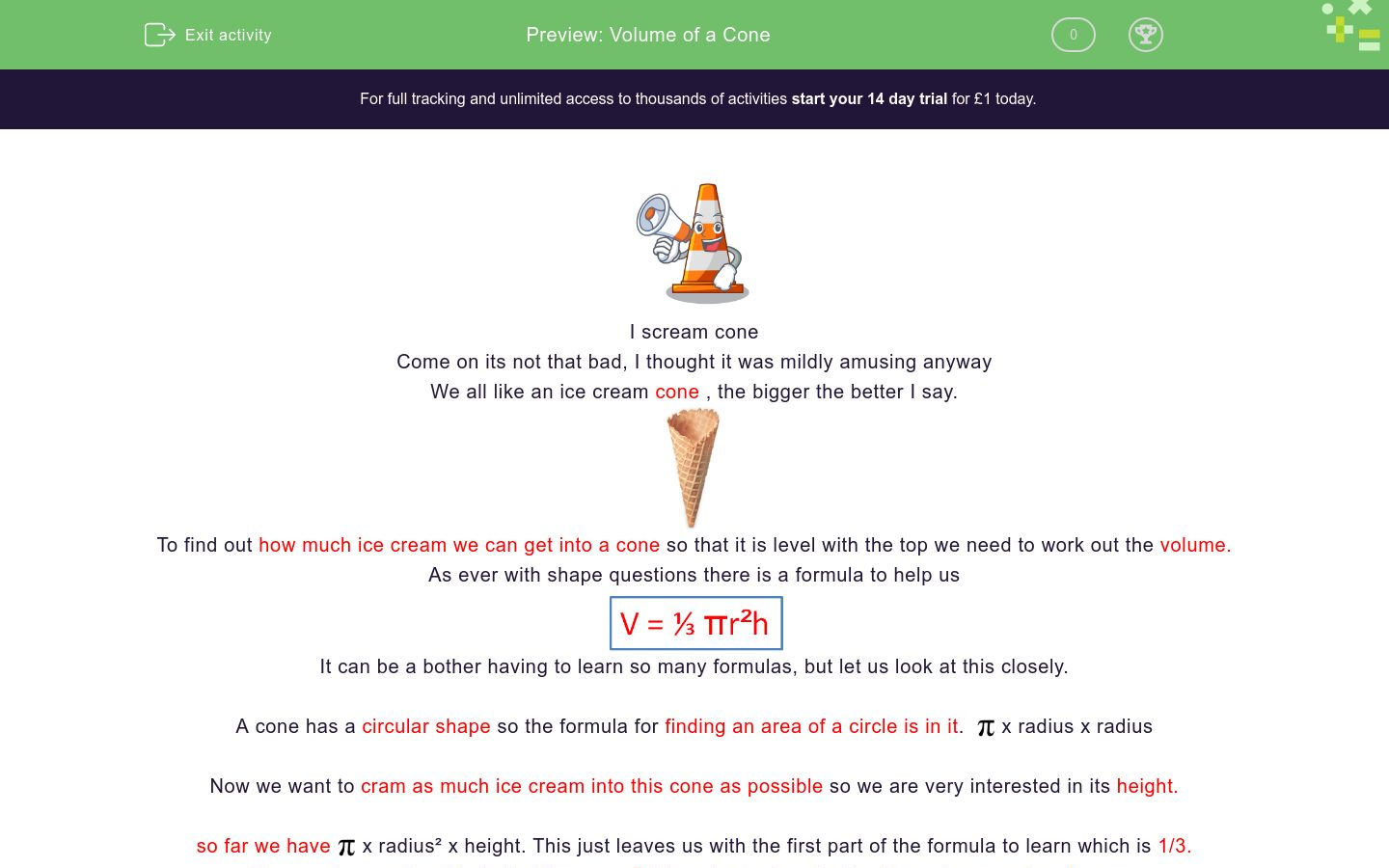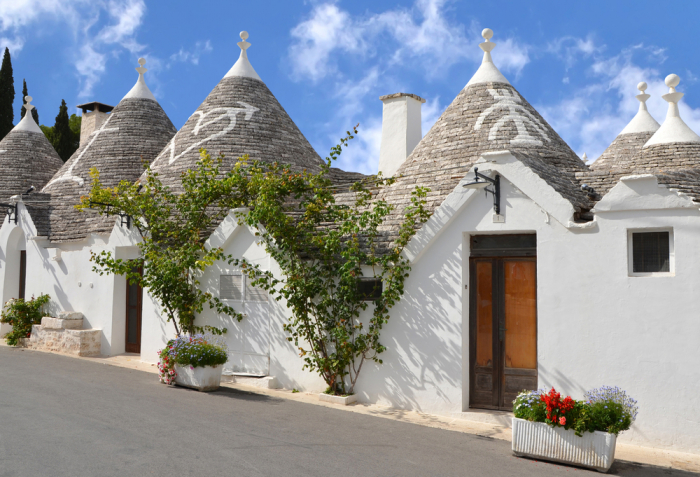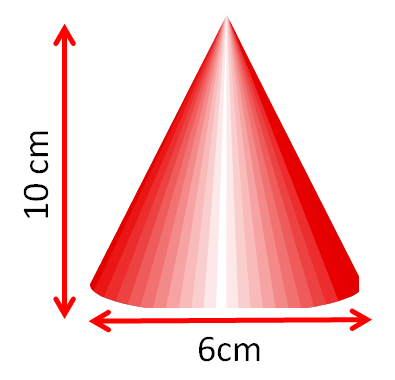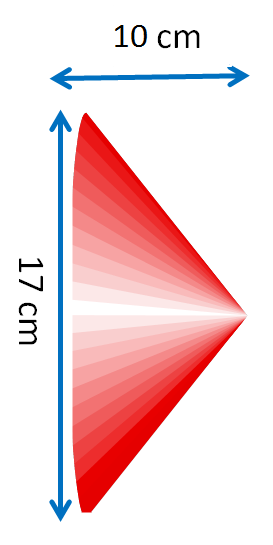# Volume of a Cone

In this worksheet students will learn the formula for finding the volume of a cone and be able to apply it.Key stage:  KS 4

GCSE Subjects:   Maths

GCSE Boards:   AQA, Eduqas, Pearson Edexcel, OCR

Curriculum topic:   Geometry and Measures, Mensuration

Curriculum subtopic:   Mensuration and Calculation, Volume and Surface Area Calculations

Difficulty level:### QUESTION 1 of 10I scream cone

Come on its not that bad, I thought it was mildly amusing anyway

We all like an ice cream cone , the bigger the better I say.To find out how much ice cream we can get into a cone so that it is level with the top we need to work out the volume.

As ever with shape questions there is a formula to help usIt can be a bother having to learn so many formulas, but let us look at this closely.

A cone has a circular shape so the formula for finding an area of a circle is in it.x radius x radius

Now we want to cram as much ice cream into this cone as possible so we are very interested in its height.

so far we havex radius² x height. This just leaves us with the first part of the formula to learn which is 1/3.

The way I remember this is that there are things to do already, I just have to remember more.

How many other examples  of a cone can you think of?Newsflash

When working with volume of more complex shapes, use thebutton on your calculator because it will useto so many decimal places.  If you use 3.142 or 3.14 answers may differ slightly.Let's get startedFind the volume of this cone.Just beware of a few things to look out for.

Sometimes you are given the diameter of the cone, remember to halve it to get the radius.

Sometimes you are given two heights, a slanted height and a vertical height. You always work with the vertical height when finding the volume.

Volume is always measure in units³

The beauty of this is just put the whole thing into you calculator in one go.

1 ÷ 3 x Π x 3²  (or 3 x 3) x 11= 103.67 cm²  rounded to 2 decimal places (2 d.p)

Can I have a vanilla cone with sprinkles on please? or do I want strawberry, or mint choc chip.....What is the formula for finding the volume of a cone?

1/3 x Π, x r², x h

1/3 x Π, x r³, x h

1/3 x Π, x r, x h

1/3 x Π, x hWhat is the volume of this party hat with a radius of 5 cm and a height of 9 cm?Cone shaped Christmas trees are all the rage these days.

What is the volume of this one? The radius is 8 cm and the height it 12 cm.

Match up the following

## Column B

Volume of a cone, radius 6 cm, height 9 cm
469 cm³
Volume of a cone, radius 7cm, height 6 cm
308 cm³
Volume of a cone, radius 8 cm, height 7 cm
201 cm³
Volume of a cone, radius 4 cm, height 12 cm
339 cm³Calculate the volume of this bamboo hat.

It has a diameter of 14 cm and a  height of 5 cm.

What is its volume?Masala dosa is a lovely meal from Southern India.

The cone is filled from a various selection of food.

Find the volume of your meal.  Radius of the cone is 7 cm and the height is 4 cm.

The answer has been rounded to 2 decimal places (2 d.p)

23.65 cm³

21.39 cm³

22.96 cm³

20.80 cm³These cone shaped roofs are found on traditional trulli houses, in the Puglia area of Italy.

Answers have been rounded to the nearest whole number.

 38 m³ 48 m³ 84 m³ 157 m³ 139 m³ 279 m³ 287 m³ Volume of roof with a radius of 3 m and a height of 4 m Volume of roof with a radius of 4 m and a height of 5 m Volume of roof with a radius of 5 m and a height of 6 m Total volumeWhat is the difference in the volume of these two cones?What is the volume of this cone?

2 cm ³

3 cm ³

4 cm ³

5 cm ³The volume of this cone is 180 cm³  height is 10 cm.

Calculate the radius of this cone?

• Question 1What is the formula for finding the volume of a cone?

1/3 x Π, x r², x h
EDDIE SAYS
Did you remember the area of a circle Π x r², together with the height. Three things going on, so you need to remember 1 out of the three 1/3. 1/3 x Π x r² x height If you did then happy days.
• Question 2What is the volume of this party hat with a radius of 5 cm and a height of 9 cm?

235.6
EDDIE SAYS
If you use the formula correctly you will never go wrong. That is why it is worth learning it. 1 ÷ 3 x Π x 5 x 5 x 9 = 235.6 cm³ Nice party hat don't you think.
• Question 3Cone shaped Christmas trees are all the rage these days.

What is the volume of this one? The radius is 8 cm and the height it 12 cm.

EDDIE SAYS
Once you know the formula it is like Christmas has come. What a gift.. 1 ÷ 3 x Π x r² x h 1 ÷ 3 x Π x 8 x 8 x 12 You just have to keep practising...
• Question 4

Match up the following

## Column B

Volume of a cone, radius 6 cm, he...
339 cm³
Volume of a cone, radius 7cm, hei...
308 cm³
Volume of a cone, radius 8 cm, he...
469 cm³
Volume of a cone, radius 4 cm, he...
201 cm³
EDDIE SAYS
Is this getting easier now? Practice makes perfect 1÷ 3 x Π x r x r x h This is all there is to it. Honestly
• Question 5Calculate the volume of this bamboo hat.

It has a diameter of 14 cm and a  height of 5 cm.

What is its volume?

256.56
EDDIE SAYS
Did you spot it? You were given the diameter so needed to halve it first to get the radius. 1 ÷ 3 x π x 7 x 7 x 5 I think i will swap my party hat for this.
• Question 6Masala dosa is a lovely meal from Southern India.

The cone is filled from a various selection of food.

Find the volume of your meal.  Radius of the cone is 7 cm and the height is 4 cm.

The answer has been rounded to 2 decimal places (2 d.p)

20.80 cm³
EDDIE SAYS
As you can see volume of cones can be applied to any situation. I don't suppose you are going to remember 1 ÷ 3 x r² x h in a restaurant though are you.
• Question 7These cone shaped roofs are found on traditional trulli houses, in the Puglia area of Italy.

Answers have been rounded to the nearest whole number.

 38 m³ 48 m³ 84 m³ 157 m³ 139 m³ 279 m³ 287 m³ Volume of roof with a radius of 3 m and a height of 4 m Volume of roof with a radius of 4 m and a height of 5 m Volume of roof with a radius of 5 m and a height of 6 m Total volume
EDDIE SAYS
Hopefully calculating the volume is second nature to you now. The added extra is knowing that total is looking for an addition at the end. I wonder what they keep in the attic of a roof shaped like that.
• Question 8What is the difference in the volume of these two cones?

662.35
EDDIE SAYS
Did you remember to halve the radius to get the diameter first? 1 ÷ 3 x Π x 3 x 3 x 10 = 94.25 1 ÷3 x Π x 8.5 x 8.5 = 756.60 Difference means subtract 756.60 - 94.25 = 662.35 cm³
• Question 9What is the volume of this cone?

3 cm ³
EDDIE SAYS
And just when you though it was safe... Don't let something like this put you off. Put this into your calculator as normal. 1 ÷ 3 x π x 3 x 3 x 1 ÷ π This is the beauty of having a scientific calculator.
• Question 10The volume of this cone is 180 cm³  height is 10 cm.

Calculate the radius of this cone?

4.15 cm
EDDIE SAYS
Really.... there is more. Don't let something like this put you off. Just use the information you have and work backwards doing the opposite mathematical calculation. Remember that 1/3 is the same as 0.3333. Again this will be easier for you. 180 ÷ 0.3333 ÷ π ÷ 10 = 17.190.... √ 17.190.. = 4.15 (2 d.p) This is the beauty of having a scientific calculator. It really is your friend at times like this.
---- OR ----

Sign up for a £1 trial so you can track and measure your child's progress on this activity.

### What is EdPlace?

We're your National Curriculum aligned online education content provider helping each child succeed in English, maths and science from year 1 to GCSE. With an EdPlace account you’ll be able to track and measure progress, helping each child achieve their best. We build confidence and attainment by personalising each child’s learning at a level that suits them.

Get started